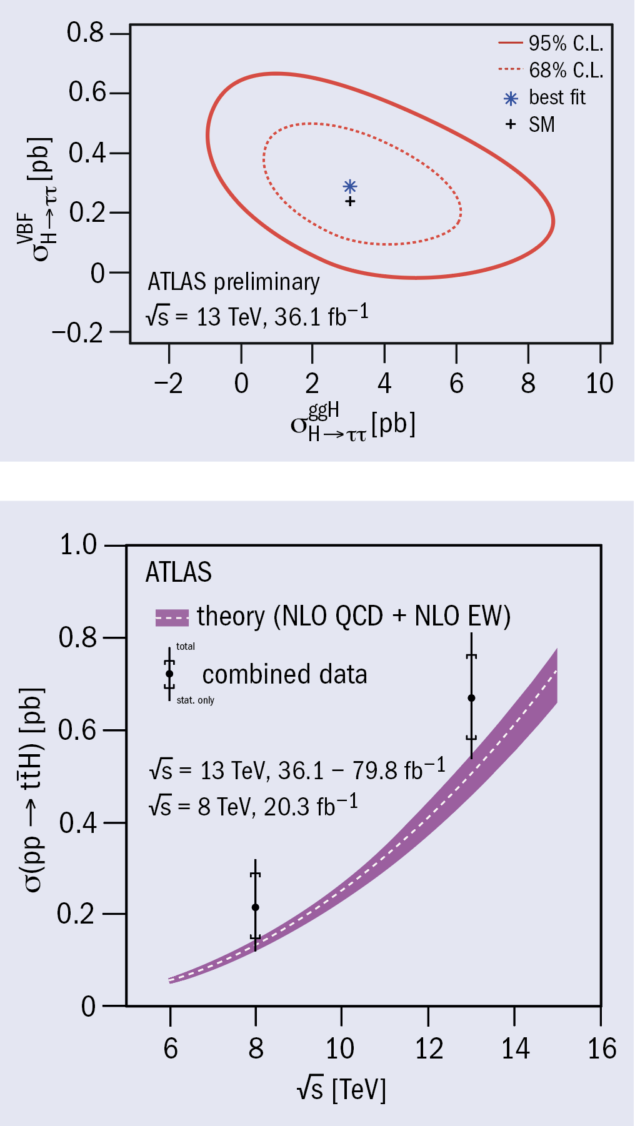# Measuring Higgs-boson interactions with third-generation fermions

9 July 2018Top: simultaneous measurement of the cross-section times branching fraction for the gluon-fusion (ggH) and vector-boson fusion (VBF) Higgs-boson production processes in Higgs-boson decays to two tau leptons. Bottom: measured ttH cross sections in pp collisions at centre-of-mass energies of 8 TeV and 13 TeV compared with the SM prediction (line with uncertainty band).

According to the Standard Model (SM), fermions acquire their mass through coupling to the Higgs field. New results released by the ATLAS collaboration firmly establish and measure these so-called Yukawa couplings to third-generation fermions. The Higgs-boson coupling to top quarks has been observed in associated production with a top quark pair (ttH production), and the Higgs-boson coupling to tau leptons has been observed in Higgs-boson decays to two tau leptons (H → ττ). Data from LHC proton–proton collisions at a centre-of-mass energy of 13 TeV recorded during 2015, 2016 and 2017 were analysed for these results.

The measurement of H → ττ, which is based on 2015 and 2016 data, was challenging because the tau lepton is short- lived and can only be observed through its decay products, of which at least one is always an invisible neutrino. The unknown momentum taken away by the neutrino makes the tau reconstruction incomplete and thus susceptible to backgrounds. Events with tau leptons are difficult to select online when the visible tau decay products are hadrons. Moreover, the Z boson, which also decays to a tau-lepton pair and is relatively close in mass to the Higgs boson but much more abundant, represents a large source of background. Good reconstruction of the di-tau invariant mass is therefore essential, using information from all detector systems to account for the missing energy.

The measured H → ττ signal has an observed (expected) statistical significance of 6.4 (5.4) standard deviations when combined with previous measurements using 7 and 8 TeV data. In 13 TeV data, the total cross-section times branching fraction was measured to be 3.71 ± 0.59 (stat) +0.87 –0.74 (syst) pb. In addition, separate measurements of the gluon fusion and weak-boson-fusion Higgs-boson production cross sections were performed (figure, top). SM predictions agree with these measurements.

The production of ttH was measured from a combination of channels involving Higgs-boson decays to a pair of W or Z bosons (WW* or ZZ*), tau leptons, b-quarks or photons. The analyses exploiting the H → γγ and H → ZZ* → 4l decays used the full 80 fb–1 proton–proton dataset collected by ATLAS between 2015 and 2017, and deployed improved reconstruction algorithms and new analysis procedures based on machine learning. The H → γγ analysis alone observed a ttH signal with a significance of 4.1 standard deviations for 3.7 expected in the SM. The H → 4l analysis expected less than one event from ttH production in the 80 fb–1 dataset and observed no event.

These results, combined with those from the other ttH channels based on 2015 and 2016 data, led to an observed (expected) significance of 5.8 (4.9) standard deviations for ttH production at 13 TeV, with a ratio of measured to predicted cross section of 1.32 . Further combination with the results from Run 1 based on data taken at 7 and 8 TeV centre-of-mass energies yielded an observed significance of 6.3 standard deviations for 5.1 expected. The measured total cross-section for ttH production at 13 TeV is 670 ± 90 (stat) ± 110 (syst) fb, in agreement with the SM prediction of 507 fb. The corresponding result at 8 TeV is 220 ± 100 (stat) ± 70 (syst) fb (figure, bottom).

With further data being collected at the LHC, more precise measurements of cross-sections and differential distributions will allow the study of the structure of Yukawa couplings in great detail and thus provide more stringent tests of the SM and increased sensitivity to physics beyond it.